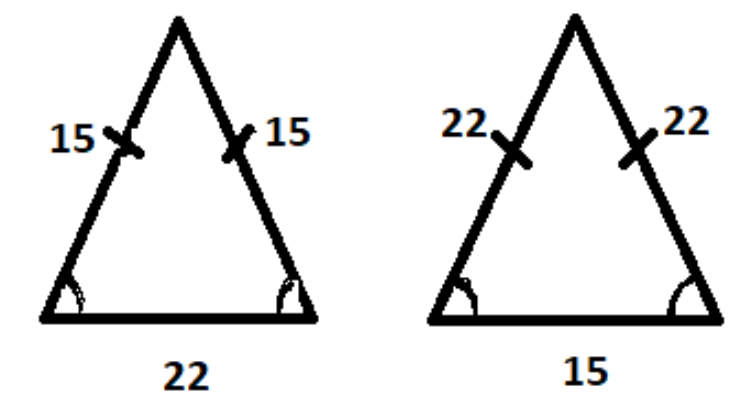QuestionAnswers

# The lengths of two sides of an isosceles triangle are $15$ and $22$, respectively, what are the possible values of perimeter?Verified
129k+ views
Hint: Here we need to apply the concept of isosceles triangle and perimeter of triangle.
Isosceles Triangle: The triangle in which the two sides of a triangle are equal, then the angles opposite to equal sides are also equal.
Perimeter of triangle = Sum of all sides.

As two sides of a triangle are given as $15$ and $22$, respectively.
We can fix either the two equal sides as $15$ and the third side is $22$ (or) the two equal sides as $22$ and the third side is $15$.
The sides of triangle can be either $15,15,22$ or $15,22,22$Perimeter of the triangle = $15+22+22=59$ or $15+15+22=52$
Therefore, the possible values of the perimeter are $59\text{ or 52}$ .